##### The Japanese Bonsai specialist
Direct order Contact Help / Services Newsletter# Root fortifying bonsai treatment 60 ml

› Bonsai soil and fertiliser › Fertilizer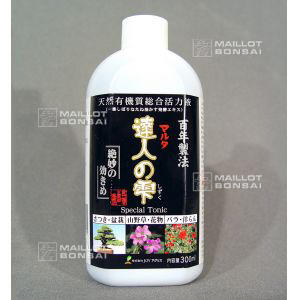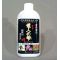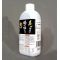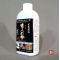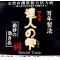ref. : 64778,60

This item is temporarily unavailableNotify availability

###### Description

Tatsujin no shizuku small bottle 60 ml. This fortifying solution contains healing amino acids to nourish bonsai and encourage root growth. It was invented by bonsai masters Katoh Saburo and Kentaro Shino to ensure the fast and balanced growth of their trees. Kentaro specialises in conifers in the Shizuoka region at the foot of Mt Fuji and has given workshops here at Maillot-Bonsaï.

Use for these species: conifers, deciduous, flowering and fruit trees.

Dosage: 10 ml per 1 litre of water. Water once a month during the growing period from May to September for outdoor bonsai and throughout the year for indoor trees. For weak specimens double the dose to 20 ml per 1 litre. For perennial plants and mini bonsai use 5ml per 1 litre.

This product cannot replace a good fertiliser, it complements it by aiding root growth.

#bonsai 7.6 #root 4.4 #fortifying 4 #growth 3.6 #litre 3.5 #trees 3.5 #fertilizer 3 #treatment 2.9 #conifers 2.8 #kentaro 2.7

Formule
(( ROUND((CHAR_LENGTH(b.article_nom)-CHAR_LENGTH(REPLACE(b.article_nom, 'bonsai', '')))/LENGTH('bonsai')) + ROUND((CHAR_LENGTH(b.article_description)-CHAR_LENGTH(REPLACE(b.article_description, 'bonsai', '')))/LENGTH('bonsai')) ) * 7.6) + (( ROUND((CHAR_LENGTH(b.article_nom)-CHAR_LENGTH(REPLACE(b.article_nom, 'root', '')))/LENGTH('root')) + ROUND((CHAR_LENGTH(b.article_description)-CHAR_LENGTH(REPLACE(b.article_description, 'root', '')))/LENGTH('root')) ) * 4.4) + (( ROUND((CHAR_LENGTH(b.article_nom)-CHAR_LENGTH(REPLACE(b.article_nom, 'fortifying', '')))/LENGTH('fortifying')) + ROUND((CHAR_LENGTH(b.article_description)-CHAR_LENGTH(REPLACE(b.article_description, 'fortifying', '')))/LENGTH('fortifying')) ) * 4) + (( ROUND((CHAR_LENGTH(b.article_nom)-CHAR_LENGTH(REPLACE(b.article_nom, 'growth', '')))/LENGTH('growth')) + ROUND((CHAR_LENGTH(b.article_description)-CHAR_LENGTH(REPLACE(b.article_description, 'growth', '')))/LENGTH('growth')) ) * 3.6) + (( ROUND((CHAR_LENGTH(b.article_nom)-CHAR_LENGTH(REPLACE(b.article_nom, 'litre', '')))/LENGTH('litre')) + ROUND((CHAR_LENGTH(b.article_description)-CHAR_LENGTH(REPLACE(b.article_description, 'litre', '')))/LENGTH('litre')) ) * 3.5) + (( ROUND((CHAR_LENGTH(b.article_nom)-CHAR_LENGTH(REPLACE(b.article_nom, 'trees', '')))/LENGTH('trees')) + ROUND((CHAR_LENGTH(b.article_description)-CHAR_LENGTH(REPLACE(b.article_description, 'trees', '')))/LENGTH('trees')) ) * 3.5) + (( ROUND((CHAR_LENGTH(b.article_nom)-CHAR_LENGTH(REPLACE(b.article_nom, 'treatment', '')))/LENGTH('treatment')) + ROUND((CHAR_LENGTH(b.article_description)-CHAR_LENGTH(REPLACE(b.article_description, 'treatment', '')))/LENGTH('treatment')) ) * 2.9) + (( ROUND((CHAR_LENGTH(b.article_nom)-CHAR_LENGTH(REPLACE(b.article_nom, 'conifers', '')))/LENGTH('conifers')) + ROUND((CHAR_LENGTH(b.article_description)-CHAR_LENGTH(REPLACE(b.article_description, 'conifers', '')))/LENGTH('conifers')) ) * 2.8) + (( ROUND((CHAR_LENGTH(b.article_nom)-CHAR_LENGTH(REPLACE(b.article_nom, 'kentaro', '')))/LENGTH('kentaro')) + ROUND((CHAR_LENGTH(b.article_description)-CHAR_LENGTH(REPLACE(b.article_description, 'kentaro', '')))/LENGTH('kentaro')) ) * 2.7) + (( ROUND((CHAR_LENGTH(b.article_nom)-CHAR_LENGTH(REPLACE(b.article_nom, 'water', '')))/LENGTH('water')) + ROUND((CHAR_LENGTH(b.article_description)-CHAR_LENGTH(REPLACE(b.article_description, 'water', '')))/LENGTH('water')) ) * 2.5)

## Secure payment## Delivery

Our logistic partners :04 74 55 23 48
Pépinière MAILLOT-BONSAÏ
Le Bois Frazy
01990 RELEVANT - FRANCE
on appointment